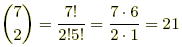index: click on a letter A B C D E F G H I J K L M N O P Q R S T U V W X Y Z A to Z index index: subject areas numbers & symbols sets, logic, proofs geometry algebra trigonometry advanced algebra & pre-calculus calculus advanced topics probability & statistics real world applications multimedia entrieswww.mathwords.com about mathwords website feedback

Binomial Coefficients in Pascal's Triangle

Numbers written in any of the ways shown below. Each notation is read aloud "n choose r".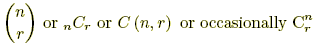These numbers, called binomial coefficients because they are used in the binomial theorem, refer to specific addresses in Pascal's triangle. They refer to the nth row, rth element in Pascal's triangle as shown below.

The formula used to compute binomial coefficients directly is found below as well.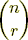refers to the nth row, rth element in Pascal's Triangle.Example: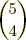is the 5th row, 4th element, so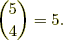5C4 is another notation for the same element.

 Formula: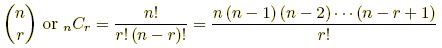Examples: